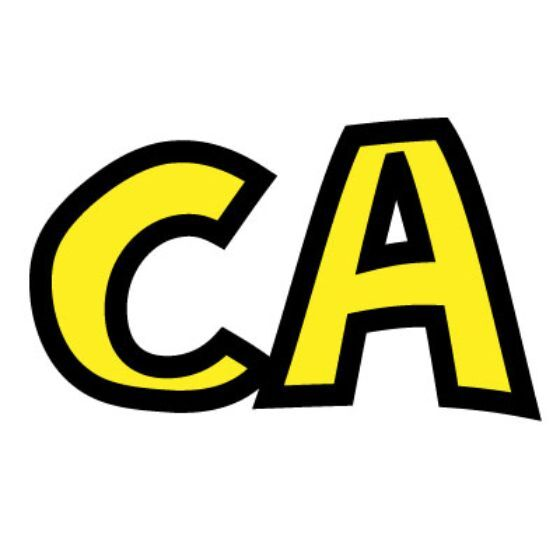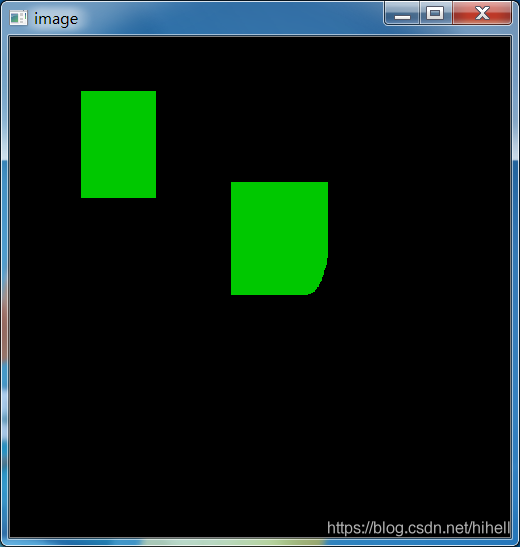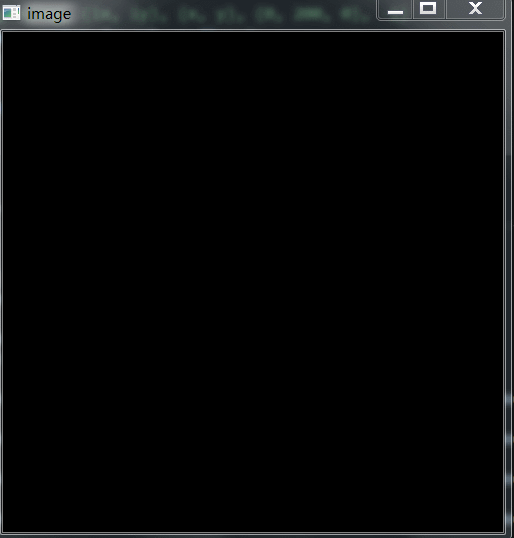# 来，在图片上画个矩形吧，取经之旅第 14 天## 使用 OpenCV 画个矩形

import cv2import numpy as np# 当鼠标按下时为 True，表示正在绘制drawing = Falseix, iy = -1, -1# 创建回调函数def draw_rect(event, x, y, flags, param):    global ix, iy, drawing    # 当按下左键时返回起始位置坐标    if event == cv2.EVENT_LBUTTONDOWN:        drawing = True        ix, iy = x, y    # 当左键按下并移动时绘制图形，event 鼠标移动状态，flag 左键拖曳状态    elif event == cv2.EVENT_MOUSEMOVE and flags == cv2.EVENT_FLAG_LBUTTON:        if drawing == True:            cv2.rectangle(img, (ix, iy), (x, y), (0, 200, 0), -1)    # 当鼠标松开时停止绘图    elif event == cv2.EVENT_LBUTTONUP:        drawing == False# 下面把回调函数与 OpenCV 窗口绑定在一起img = np.zeros((500, 500, 3), np.uint8)cv2.namedWindow('image')cv2.setMouseCallback('image', draw_rect)while(1):    cv2.imshow('image', img)    k = cv2.waitKey(1)    if k == ord('q'):        breakcv2.destroyAllWindows()img = np.zeros((500, 500, 3), np.uint8)

zeros(shape, dtype=float, order='C')

• shape：形状

• dtype：数据类型，可选参数，默认 numpy.float64

• order：可选参数，C 代表与 C 语言类似，行优先；F 代表列优先

print(np.zeros((2,5)))

[[0. 0. 0. 0. 0.] [0. 0. 0. 0. 0.]]

dst = np.zeros((height, width, 3), np.uint8)

## 使用 OpenCV 画曲线

cv2.circle(img,(x,y),3,(0,0,200),-1)## 使用 OpenCV 画直线

import cv2import numpy as npix, iy = -1, -1# 创建回调函数def draw_rect(event, x, y, flags, param):    global ix, iy    # 当按下左键时返回起始位置坐标    if event == cv2.EVENT_LBUTTONDOWN:        ix, iy = x, y    elif event == cv2.EVENT_LBUTTONUP:        cv2.line(img, (ix, iy), (x, y), (0, 255, 0), 3)print(np.zeros((500, 500, 3), np.uint8))# 下面把回调函数与 OpenCV 窗口绑定在一起img = np.zeros((500, 500, 3), np.uint8)cv2.namedWindow('image')cv2.setMouseCallback('image', draw_rect)while(1):    cv2.imshow('image', img)    k = cv2.waitKey(1)    if k == ord('q'):        breakcv2.destroyAllWindows()## OpenCV 尾声

1 个小时又过去了，对 Python OpenCV 相关的知识点，你掌握了吗？

**想学 Python 爬虫，可以订阅橡皮擦专栏哦~** 🈲🈲🈲🈲 点击发现惊喜 🈲🈲🈲🈲### 梦想橡皮擦

6 年产品经理+教学经验，3 年互联网项目管理经验； 互联网资深爱好者； 沉迷各种技术无法自拔，导致年龄被困在 25 岁； CSDN 爬虫 100 例作者。 个人公众号“梦想橡皮擦”。

## 评论